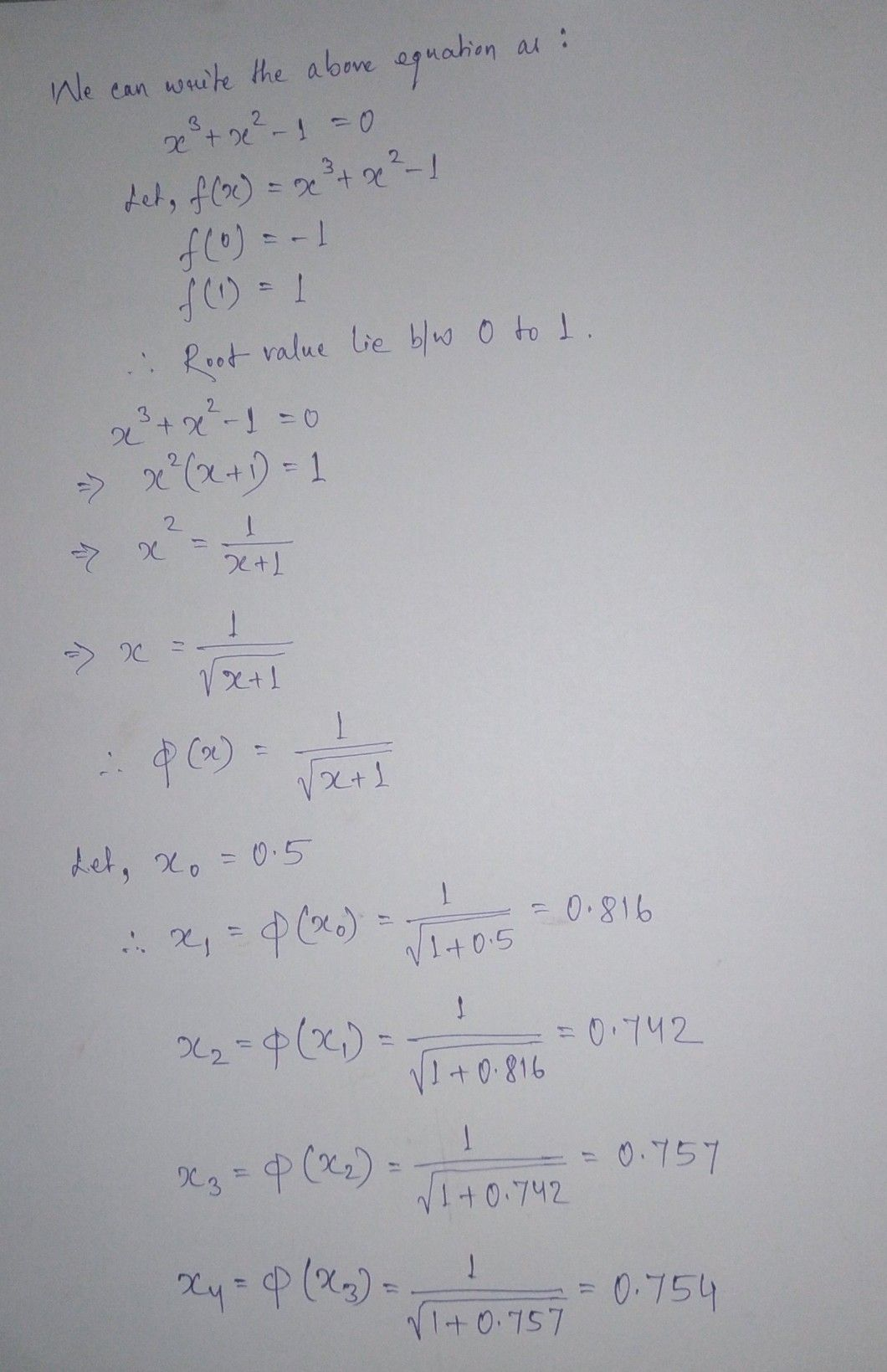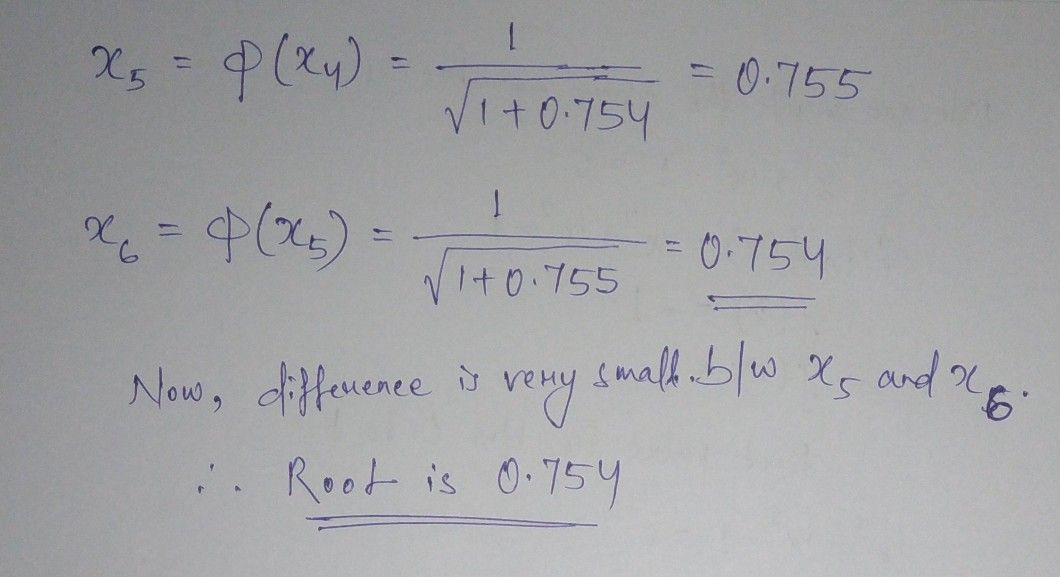Symbol
Problem$\right)$ ) Find the real root of equation $x^{3}+x^{2}-1=0$ uring iterative method correct to four decimal places.
Algebra
Search count: 107
SolutionQanda teacher - BhanuStudent
thnk uhh